# Emission

As you have observed, the light seen from hydrogen is created from transitions from one energy state to another. To explain emission, we will look at the wavelengths created, looking at the different "groups" of transitions created. One group will consist of Ultra Violet light and one group will consist of Visible light. We can group the rest of the spectrum following simila patterns.

Electrons in each group before we apply energy to the Hydrogen tube would be sitting in a common state, also called the ground state. When the tube is on, the electrons get excited and some will move to higher energies than others. We call the higher energy levels the excited states.

The energy of an electron in an atom is negative. The negative sign indicates that we need to give the electron energy to move it from the hydrogen atom. Giving the electron energy will increase the amound of energy in the atom. the electron prefers to spend mos of its time in a lower energy level, just as a ball on Earth prefers to rest in valleys instead of hills. The electron stays in an excited state for a short time. When the electron transits from an excited state to its lower energy state, it will gice off the same amound of energy needed to raise to that level. This emitted energy is a photon. The energy of the photon will determine the color of the Hydrogen Spectra seen.

Knowing the photon's energy, we can use our equation from Planck.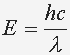to find the wavelenth of light.

The hydrogen atom is a single electron atom. It has one electron attached to the nucleus. The energy in a hydrogen atom depends on the energy of the electron. When the electron changes levels, it decreases energy and the atom emits photons. The photon is emitted with the electron moving from a higher energy level to a lower energy level. The energy of the photon is the exact energy that is lost by the electron moving to its lower energy level.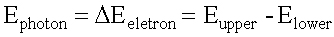When the electron changes from n=3 or above to n=2, the photons emitted fall in the Visible Light region of the spectra. We call these lines Balmer's Series.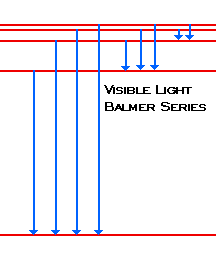The relation of the energy levels and wavelength is: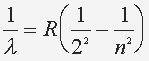where n=3,4,5 ... and R = 1.097 x 10^7 (1/m) When the electron changes from n=2 or above to n=1, the photons emitted fall in the Ultra Violet region of the spectra. We call these lines Lyman's Series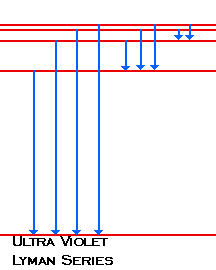The relation of the energy levels and wavelength is: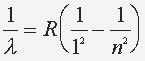where n=2,3,4 ... and R = 1.097 x 10^7 (1/m)

In the infrared region, we have Paschen, Brackett, and Pfund series which are transitions from high levels to n=3, n=4, and n=5 respectivly.

The R in the equation is the Rhydberg Constant. The newest up-to-date value for the Rhydberg constant can be found at Fundamental Physical Constants - NIST and search for "Rydberg constant".